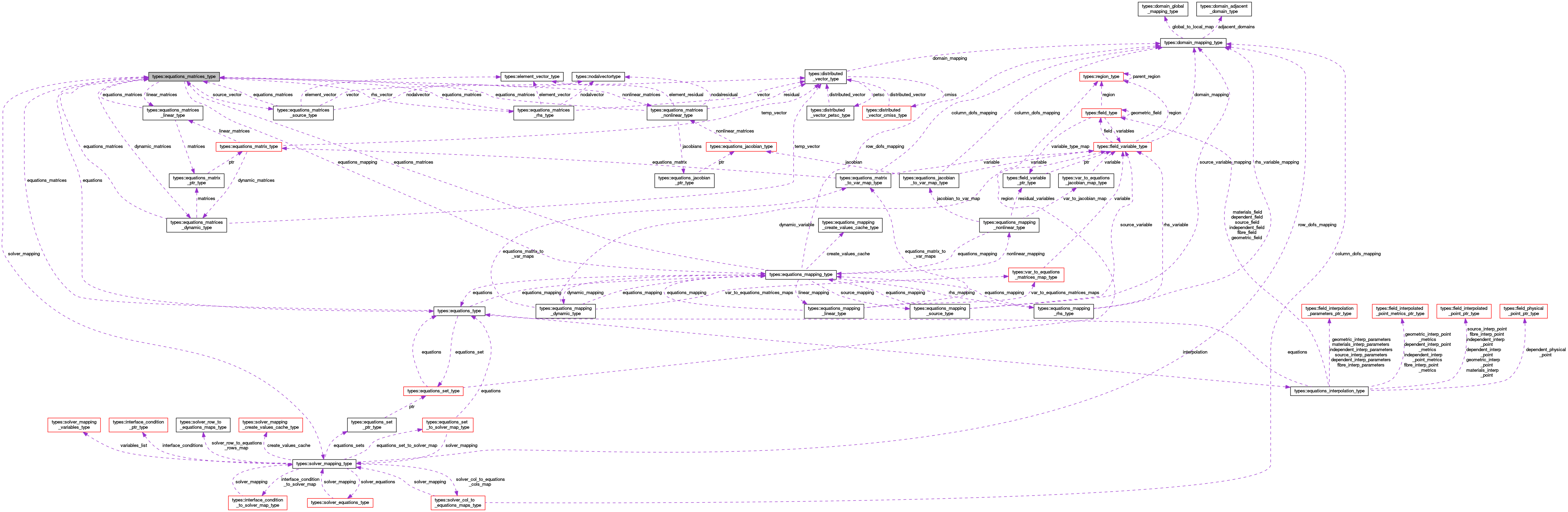OpenCMISS-Iron Internal API Documentation
types::equations_matrices_type Type Reference

Contains information on the equations matrices and vectors. More...

Collaboration diagram for types::equations_matrices_type:[legend]

## Public Attributes

type(equations_type), pointer equations
A pointer back to the equations. More...

logical equations_matrices_finished
Is .TRUE. if the equations matrices have finished being created, .FALSE. if not. More...

type(equations_mapping_type), pointer equations_mapping
A pointer to the equations mapping for the equations matrices. More...

type(solver_mapping_type), pointer solver_mapping
A pointer to the solver mapping for the equations matrices. More...

integer(intg) number_of_rows
The number of local rows (excluding ghost rows) in the distributed equations matrices and vectors. More...

integer(intg) total_number_of_rows
The number of local rows (including ghost rows) in the distributed equations matrices and vectors. More...

integer(intg) number_of_global_rows
The number of global rows in the distributed equations matrices and vectors. More...

type(equations_matrices_dynamic_type), pointer dynamic_matrices
A pointer to the dynamic matrices information for the equations matrices. More...

type(equations_matrices_linear_type), pointer linear_matrices
A pointer to the linear matrices information for the equations matrices. More...

type(equations_matrices_nonlinear_type), pointer nonlinear_matrices
A pointer to the nonlinear matrices and vectors information for the equations matrices. More...

type(equations_matrices_rhs_type), pointer rhs_vector
A pointer to the RHS vector information for the equations matrices. More...

type(equations_matrices_source_type), pointer source_vector
A pointer to the source vector information for the equations matrices. More...

## Detailed Description

Contains information on the equations matrices and vectors.

Definition at line 1520 of file types.f90.

## Member Data Documentation

 type(equations_matrices_dynamic_type), pointer types::equations_matrices_type::dynamic_matrices

A pointer to the dynamic matrices information for the equations matrices.

Definition at line 1529 of file types.f90.

 type(equations_type), pointer types::equations_matrices_type::equations

A pointer back to the equations.

Definition at line 1521 of file types.f90.

 type(equations_mapping_type), pointer types::equations_matrices_type::equations_mapping

A pointer to the equations mapping for the equations matrices.

Definition at line 1523 of file types.f90.

 logical types::equations_matrices_type::equations_matrices_finished

Is .TRUE. if the equations matrices have finished being created, .FALSE. if not.

Definition at line 1522 of file types.f90.

 type(equations_matrices_linear_type), pointer types::equations_matrices_type::linear_matrices

A pointer to the linear matrices information for the equations matrices.

Definition at line 1530 of file types.f90.

 type(equations_matrices_nonlinear_type), pointer types::equations_matrices_type::nonlinear_matrices

A pointer to the nonlinear matrices and vectors information for the equations matrices.

Definition at line 1531 of file types.f90.

 integer(intg) types::equations_matrices_type::number_of_global_rows

The number of global rows in the distributed equations matrices and vectors.

Definition at line 1527 of file types.f90.

 integer(intg) types::equations_matrices_type::number_of_rows

The number of local rows (excluding ghost rows) in the distributed equations matrices and vectors.

Definition at line 1525 of file types.f90.

 type(equations_matrices_rhs_type), pointer types::equations_matrices_type::rhs_vector

A pointer to the RHS vector information for the equations matrices.

Definition at line 1532 of file types.f90.

 type(solver_mapping_type), pointer types::equations_matrices_type::solver_mapping

A pointer to the solver mapping for the equations matrices.

Definition at line 1524 of file types.f90.

 type(equations_matrices_source_type), pointer types::equations_matrices_type::source_vector

A pointer to the source vector information for the equations matrices.

Definition at line 1533 of file types.f90.

 integer(intg) types::equations_matrices_type::total_number_of_rows

The number of local rows (including ghost rows) in the distributed equations matrices and vectors.

Definition at line 1526 of file types.f90.